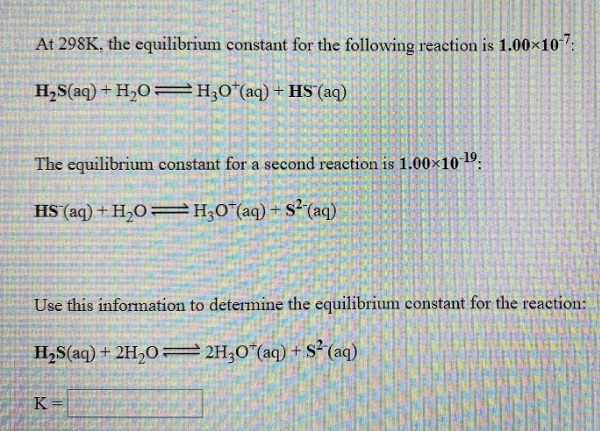# At 298K, the equilibrium constant for the following reaction is 1.00x10^-7: H2S(aq) + H2O ⇌ H3O^+(aq) + HS^-(aq) The equilibrium constant for a second reaction is 1.00x10^-19: HS^-(aq) + H2O ⇌ H3O^+(aq) + S^2-(aq) Use this information to determine the equilibrium constant for the reaction: H2S(aq) + 2H2O ⇌ 2H3O^+(aq) + S^2-(aq)